# Test: Molecular Orbital Theory (NCERT)

## 15 Questions MCQ Test Topic-wise MCQ Tests for NEET | Test: Molecular Orbital Theory (NCERT)

Description
Attempt Test: Molecular Orbital Theory (NCERT) | 15 questions in 15 minutes | Mock test for NEET preparation | Free important questions MCQ to study Topic-wise MCQ Tests for NEET for NEET Exam | Download free PDF with solutions
QUESTION: 1

### Which of the following statements is not true regarding molecular orbital theory?

Solution:

Bonding molecular orbital has lower energy than the antibonding molecularorbital.

QUESTION: 2

### 2s and 2p-atomic orbitals combine to give how many molecular orbitals?

Solution:

2s-> (σ2s) (0*2s) (2)

2p -> (σ2pz) (σ*2pz)(π2px)(π*2px) (π2py) (π*2py)  (6)

QUESTION: 3

### The conditions for the combination of atomic orbitals to form molecular orbitals are stated below. Mark the incorrect condition mentioned here.

Solution:

Sigma molecular orbitals are symmetrical around the bond axis while pi molecular orbitals are not symmetrical.

QUESTION: 4

The electronic configuration of carbon is Is2 2s2 2p2. There are 12 electrons in C2. The correct electronic configuration of C2 molecule is

Solution:

For atoms like nitrogen and below N in atomic number have higher energy for pz -orbital, hence the configuration for C2 molecule is

(σls2)(σ*1s2) (σ2s2) (σ*2s2) (π2p2= π2p2y)

QUESTION: 5

The increasing order of energies of various molecular orbitals of N2 is given below:
σls <σ*ls <σ2s <σ*2s <π2px = π2py <σ2pz < π*2px = π*2py<σ*2pz

The above sequence is not true for the molecule

Solution:

For allel ements which have atomic number more than 7(beyond nitrogen) the energy of σ2pz is lower than
π2px  and π2py -orbitals.

QUESTION: 6

Which of the following species has unpaired electrons?

Solution: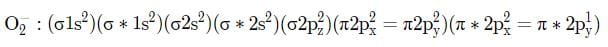N2, F2 and O2-have paired electrons

QUESTION: 7

What is the order of stability of N2 and its ions?

Solution:

Bond order of N2 = 3, N+2 = 2.5 N-2 = 2.5 and N22- is 2. Higher the bond order, more is the stability.

QUESTION: 8

What will be the bond order of the species with electronic configuration 1s2s2 2p5?

Solution:

Electronic configuration of atom: 1s2s2 2p5
M.O. configuration: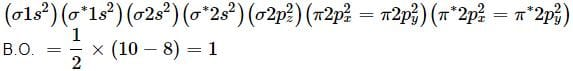QUESTION: 9

Which of the following bond orders is indication of existence of a molecule?

Solution:

A molecule exists only if the bond order is positive. If bond order is zero or negative, the molecule does not exist.

QUESTION: 10

Which of the following pairs will have same bond order?

Solution:

F2 and O2-2  are isoelectronic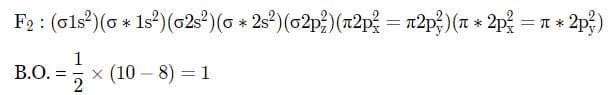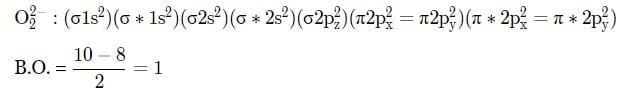QUESTION: 11

Bond order of N+2,N-2 and N2 will be

Solution: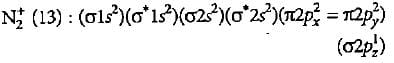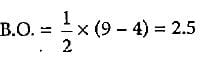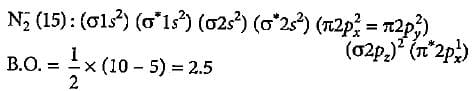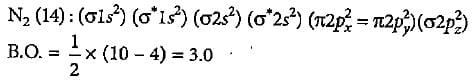QUESTION: 12

According to molecular orbital theory, which of the following will not exist?

Solution:

Be2: (σ1s2)  (σ*1s2) (σ2s2) (σ*2s2)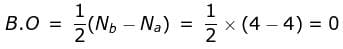Since B.O. is zero, the molecule does not exist.

QUESTION: 13

Which of the following facts regarding bond order is not valid?

Solution:

Higher the bond order, higher is the bond enthalpy.

QUESTION: 14

Which of the following formulae does not show the correct relationship?

Solution:

Bond order ∝ Bond dissociation energy.

QUESTION: 15

Fill in the blanks with the appropriate choice.
Bond order of N+2  is ___P___  while that of N2 is ___Q___ . Bond order of O+ is ___R___  while that of O2  is ___S___ . N−N bond distance ___T___ , when N2 changes to N+and when O2 changes to O+2 , the O-O bond distance ___U___ .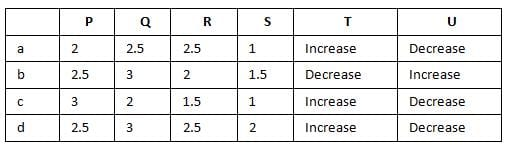Solution: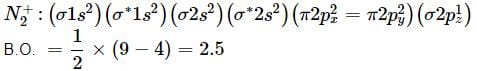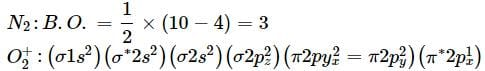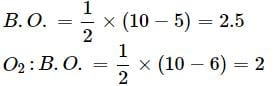Since N+2 has lower bond order than N2, bond length of N - N in N+2  increases .In O+2 , bond order increases form 2 to 2.5 hence , bond length decreases.Use Code STAYHOME200 and get INR 200 additional OFF Use Coupon Code